﻿ 多参数约束磁性体三维形态反演
 石油地球物理勘探2021, Vol. 56Issue (2): 407-418  DOI: 10.13810/j.cnki.issn.1000-7210.2021.02.0240文章快速检索 高级检索

### 引用本文LI Jinpeng, FAN Hongbo, LIU Li, ZHANG Yingtang, LI Zhining, WU Bingyang. Multi-parameter constrained three-dimensional shape inversion of magnetic bodies. Oil Geophysical Prospecting, 2021, 56(2): 407-418. DOI: 10.13810/j.cnki.issn.1000-7210.2021.02.024.### 作者简介范红波, 河北省石家庄市新华区和平西路97号陆军工程大学石家庄校区, 050003。Email: ffhhbboo@163.com

### 文章历史

① 93114部队, 北京 100195;
② 陆军工程大学石家庄校区, 河北石家庄 050003;
③ 北京市遥感信息研究所, 北京 100192

Multi-parameter constrained three-dimensional shape inversion of magnetic bodies
LI Jinpeng , FAN Hongbo, LIU Li , ZHANG Yingtang , LI Zhining , WU Bingyang
① Unit 93114 of PLA, Beijing 100195, China;
② Army Engineering University(Shijiazhuang), Shijiazhuang, Hebei 050003, China;
③ Beijing Institute of Remote Sensoring Information, Beijing 100192, China
Abstract: Traditionally, the magnetic and geometric parameters for shape inversion of magnetic bodies are defined manually. We propose a three-dimensional shape inversion method of magnetic bodies based on multi-parameter constraints. First, an objective function is established through L1 norm and regularization constraint function, and the optimal solution is selected in the module to be updated to update the inversion model; then the method for estimating the multiple parameters of the magnetic target is proposed, which can directly estimate the position, magnetization direction and magnetization intensity of the magnetic target without prior information; and finally, the magnetic component (Bz) along z and magnetic gradient tensor are used for joint inversion, and the inversion result is compared with normalized source strength and total magnitude. The simulation and experimental results show that, even without prior information, the method we proposed can effectively estimate the magnetic parameters of the magnetic target and accurately obtain the inversion results of the magne-tic target. At the same time, under the condition of remanent magnetization, compared with the traditional normalized source strength and total magnitude, this method can effectively improve the accuracy of three-dimensional inversion of magnetic bodies.
Keywords: three-dimensional shape inversion    multi-parameter    magnetic target    prior information
0 引言

1 方法原理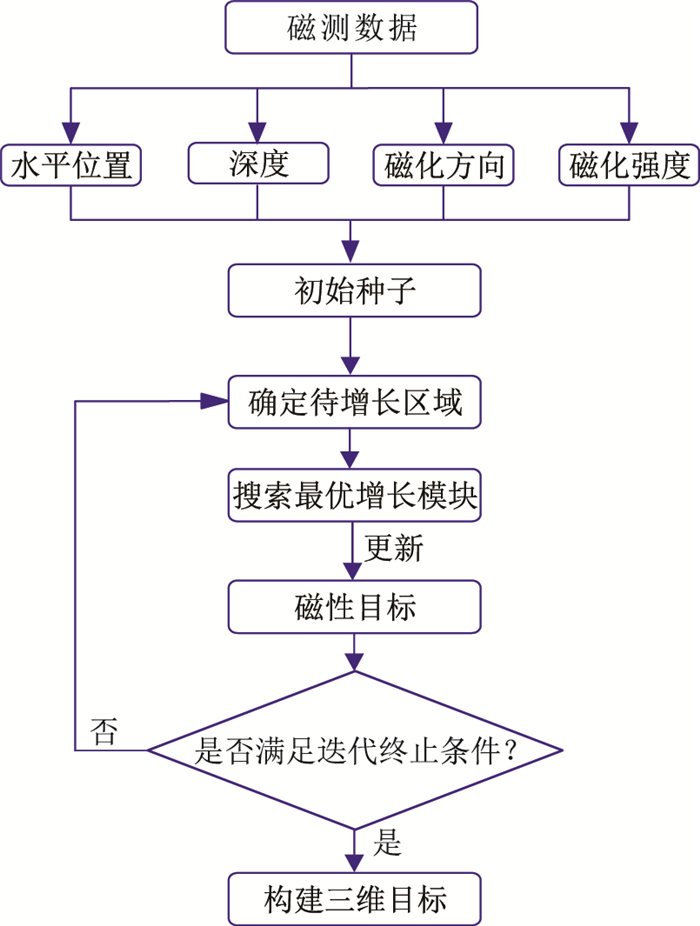图 1 本文算法流程图
1.1 形态反演理论

 $\phi=\frac{\sum\limits_{i=1}^{N}\left|d_{i}-b_{i}\right|}{\sum\limits_{i=1}^{N}\left|d_{i}\right|}$ (1)

 $\boldsymbol{B}=\left[\begin{array}{c} \frac{\partial}{\partial x} \\ \frac{\partial}{\partial y} \\ \frac{\partial}{\partial z} \end{array}\right]\left[\begin{array}{lll} B_{x} & B_{y} & B_{z} \end{array}\right]=\left[\begin{array}{lll} B_{x x} & B_{x y} & B_{x z} \\ B_{y x} & B_{y y} & B_{y z} \\ B_{z x} & B_{z y} & B_{z z} \end{array}\right]$ (2)

 $\varPsi(M)=\sum\limits_{l=1}^{H} \phi_{l}(M)$ (3)

 $\varGamma(M)=\varPsi(M)+\rho \theta(M)$ (4)

 $\rho^{(n+1)}=q \rho^{(n)}$ (5)

 $\theta(M)=\frac{1}{w+f+g} \sum\limits_{j=1}^{P} \frac{M_{j}}{M_{j}+\varepsilon} L_{j}$ (6)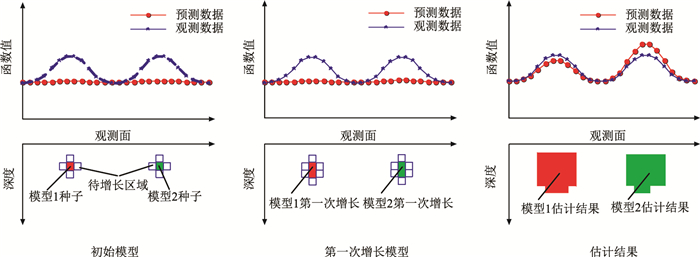图 2 形态反演模型增长示意图
1.2 磁性目标多参数反演

1.2.1 平面位置估计

 \left\{\begin{aligned} A_{1}=& B_{x x} B_{y y}+B_{y y} B_{z z}+B_{x x} B_{z x}-\\ & B_{x y}^{2}-B_{y z}^{2}-B_{x z}^{2} \\ A_{2}=& B_{x x}\left(B_{y y} B_{z z}-B_{y z}^{2}\right)+B_{x y}\left(B_{y z} B_{x z}-\right.\\ &\left.B_{x y} B_{z z}\right)+B_{x z}\left(B_{x y} B_{y z}-B_{x z} B_{y y}\right) \\ \mathrm{NSS}&= \sqrt{-\lambda_{2}^{2}-\lambda_{1} \lambda_{3}} \end{aligned}\right. (7)

1.2.2 深度估计

 $\mathrm{F}\left(B_{z}\right)=\left[\frac{\mu_{0}}{2} \gamma_{m} \mathrm{e}^{k z_{0}}\left(\mathrm{e}^{-k z_{1}}-\mathrm{e}^{-k z_{2}}\right)\right] \times \mathrm{F}(M)$ (8)

 $\mathrm{F}\left(M_{c}\right)=\frac{2}{\mu_{0} \gamma_{m}} \times \frac{h\left(k_{x}, k_{y}, z_{c}\right)}{\int_{z=z_{0}}^{\infty} h\left(k_{x}, k_{y}, z\right) \times \mathrm{e}^{-k x} \mathrm{~d} z} \times \mathrm{F}\left(B_{z}\right)$ (9)

1.2.3 磁化方向估计

 $Q=\frac{\sum\limits_{u=1}^{N_{x}} \sum\limits_{v=1}^{N_{y}}\left[\Delta T_{\mathrm{rtp}}(u, v)-\overline{\Delta T_{\mathrm{rtp}}}\right] \times[\mathrm{NSS}(u, v)-\overline{\mathrm{NSS}}]}{\sqrt{\sum\limits_{u=1}^{N_{x}} \sum\limits_{v=1}^{N_{y}}\left[\Delta T_{\mathrm{rtp}}(u, v)-\overline{\Delta T_{\mathrm{rtp}}}\right]^{2} \times[\mathrm{NSS}(u, v)-\overline{\mathrm{NSS}}]^{2}}}$ (10)

1.2.4 磁化强度估计

(1) 估计磁性目标初始种子的位置及磁化方向信息，给定初始磁化强度，并对磁性目标进行反演。

(2) 根据步骤(1)的反演结果，结合磁性目标的位置信息及磁化方向信息，将磁化强度按照一定的间隔，取一系列值，并按照从小到大的顺序进行正演计算。

(3) 定义均方根误差

 $\mathrm{RMSE}=\sqrt{\frac{1}{N_{x} N_{y} }\sum\limits_{u=1}^{N_{x}} \sum\limits_{v=1}^{N_{y}}[b(u, v)-d(u, v)]^{2}}$

(4) 重复步骤(1)~步骤(3)，并将初始磁化强度定义为上一次计算得到的最优磁化强度值，直到RMSE小于预设的精度或者达到预设的迭代次数。

1.3 形态反演迭代终止准则

 $\eta(M)=\frac{\left|\varPhi_{\text {new }}(M)-\varPhi_{\text {old }}(M)\right|}{\left|\varPhi_{\text {old }}(M)\right|}$ (11)

2 仿真分析 2.1 长方体模型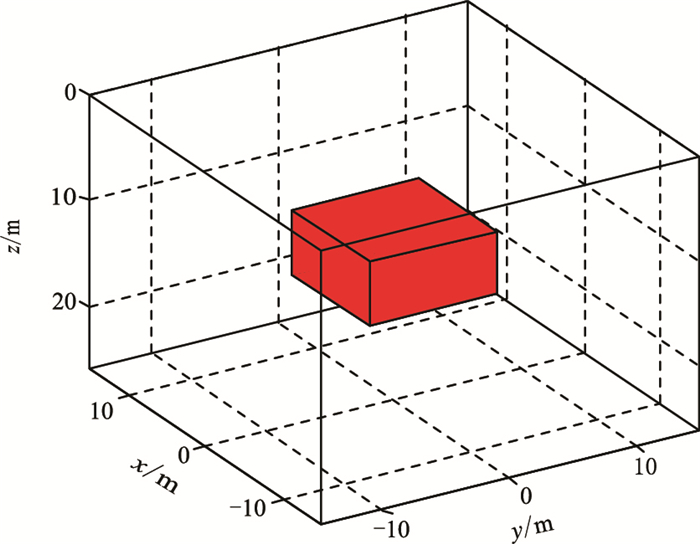图 3 长方体模型示意图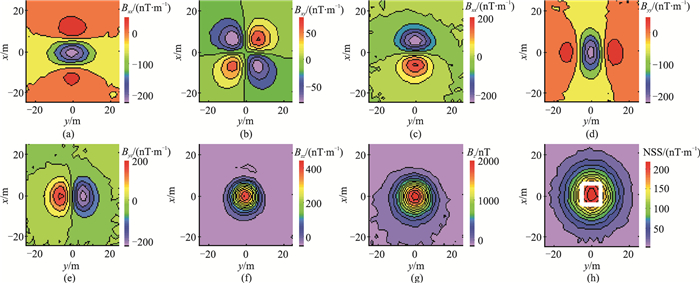图 4 长方体模型加噪磁张量及NSS计算结果 (a)Bxx；(b) Bxy；(c)Bxz；(d)Byy；(e)Byz；(f)Bzz；(g)Bz；(h)NSS(白线方框为磁性体的水平位置)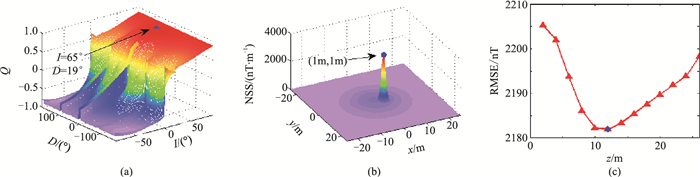图 5 长方体模型参数估计结果 (a)磁化方向；(b)水平位置；(c)深度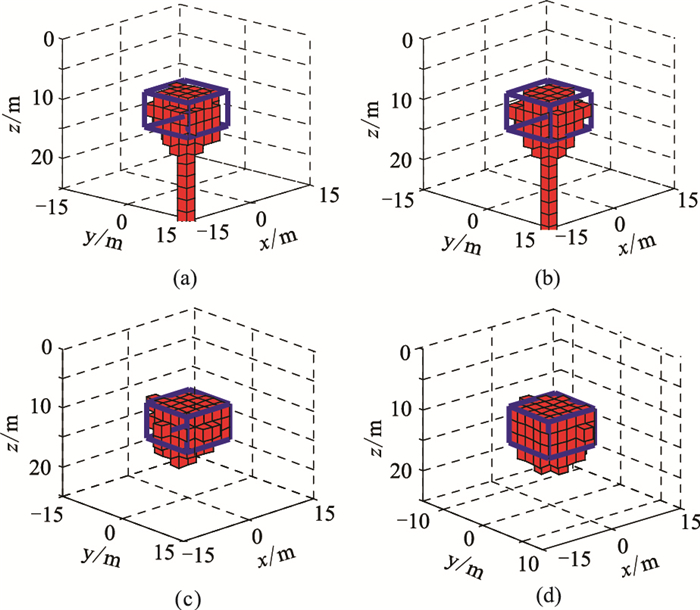图 6 不同数据组合条件下的磁性体形态反演结果 (a)Bzz；(b) Bz/Bxx/Bxy/Bxz/Byy/Byz/Bzz；(c)Bz/Bzz；(d)Bz/Bxx/Bxy/Bxz/Byy/Byz/Bzz。蓝色轮廓表示异常体边界，红色块体表示反演模型，图 7、图 11、图 12、图 16、图 20同表 1 不同数据组合条件下反演结果的正演数据RMSE统计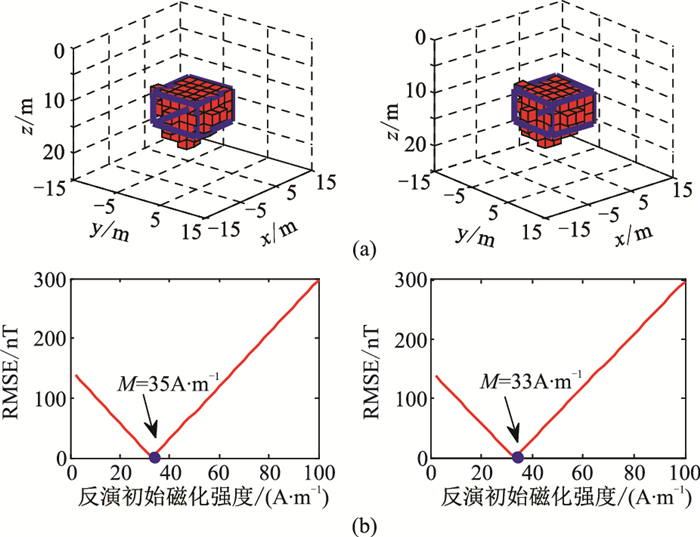图 7 初始磁化强度为1A/m(左)和200A/m(右)条件下模型三维反演结果(a)及设定不同初始磁化强度下反演模型的正演误差(b)
2.2 倾斜板状体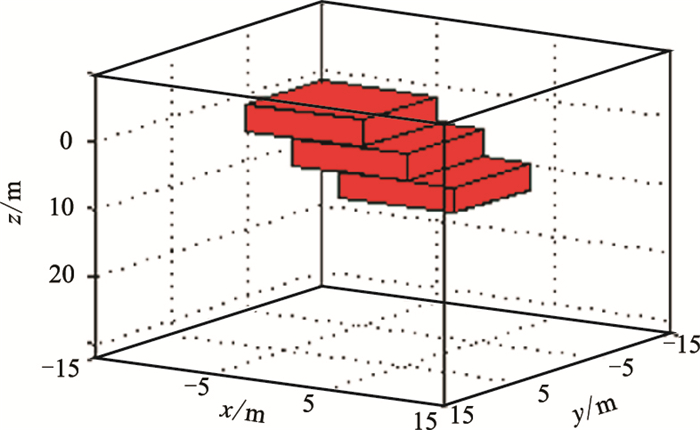图 8 倾斜板状磁性体模型空间示意图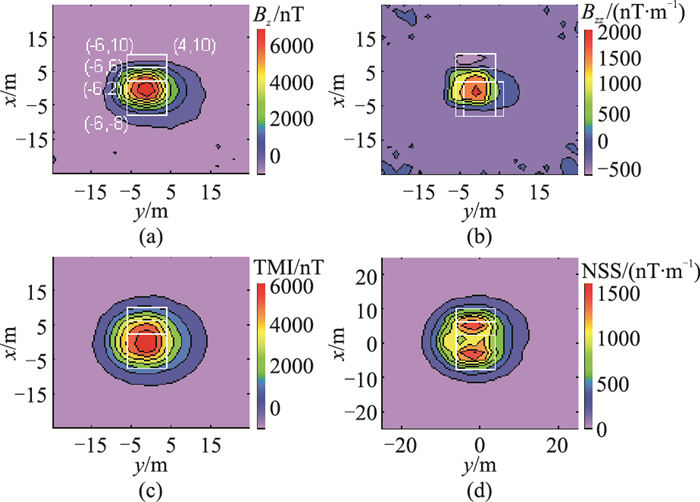图 9 倾斜板状磁性体模型正演磁测数据(加噪) (a)Bz；(b)Bzz；(c)TMI；(d)NSS。图中白线为磁性目标水平位置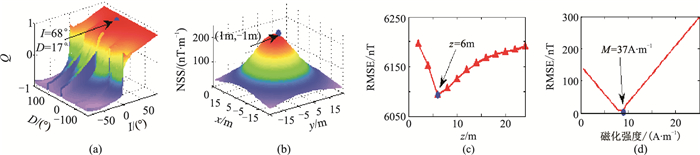图 10 倾斜板状磁性体模型本文方法参数估计结果 (a) 磁化方向；(b)水平位置；(c)深度；(d) 磁化强度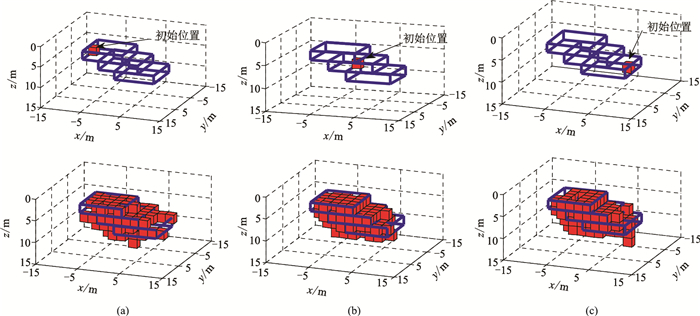图 11 倾斜板状磁性体模型种子点初始位置1(a)、2(b)、3(c)的反演结果 上图为种子点位置，下图为对应的反演结果表 2 不同初始位置条件下不同数据组合反演模型的不同参数正演结果RMSE统计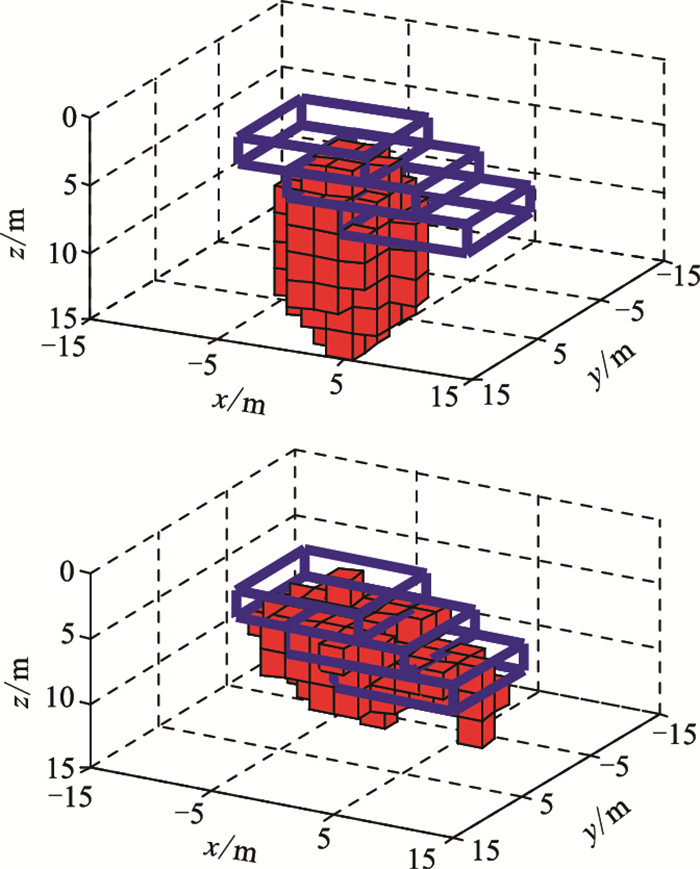图 12 倾斜板状磁性体模型NSS(上)和TMI(下)反演结果
2.3 多磁性体模型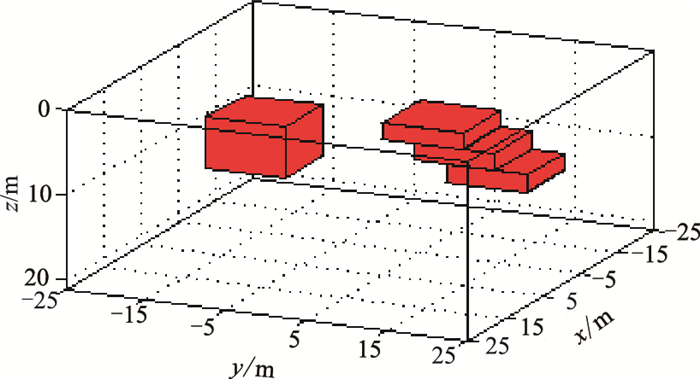图 13 组合模型示意图表 3 多磁性体模型参数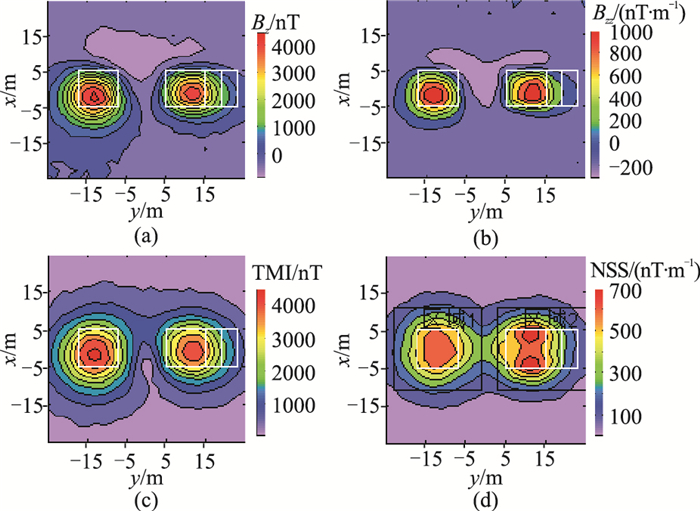图 14 组合模型磁测数据 (a)Bz；(b)Bzz；(c)TMI；(d)NSS。图中白色方框为磁性目标体实际水平范围，黑色方框为划定的反演区域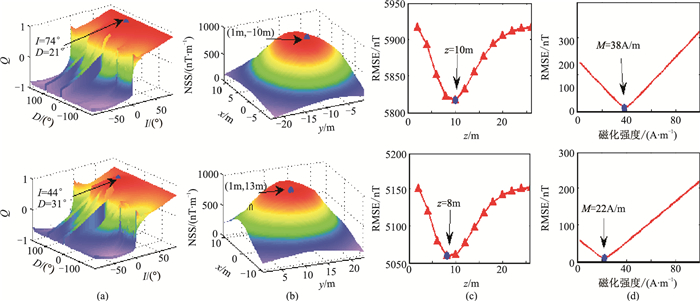图 15 利用本文方法得到的区域1(上)和区域2(下)的磁化方向(a)、水平位置(b)、深度(c)和磁化强度(d)估计结果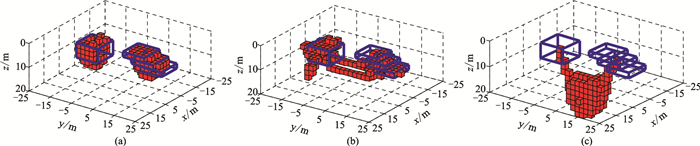图 16 剩磁条件下利用Bz/Bzz(a)、TMI(b)和NSS(c)反演的多目标体模型空间形态
3 试验验证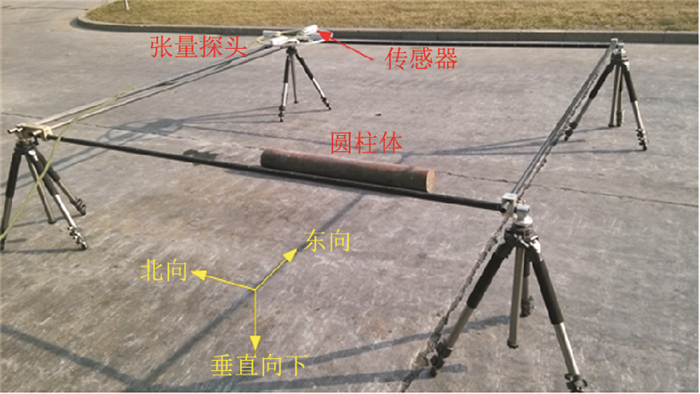图 17 试验装置及待测模型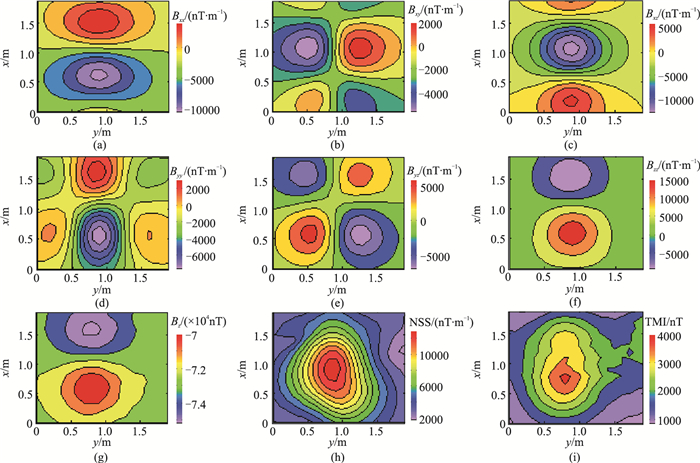图 18 实验实测数据 (a)Bxx；(b) Bxy；(c)Bxz；(d)Byy；(e) Byz；(f)Bzz；(g)Bz；(h)NSS；(i)TMI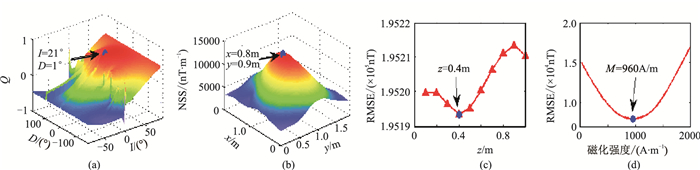图 19 基于实测数据的模型参数估计结果 (a)磁化方向；(b)水平位置；(c)深度；(d)磁化强度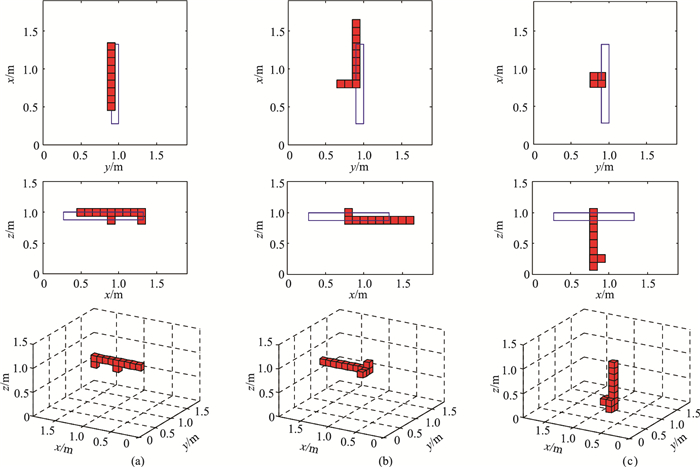图 20 基于Bz/Bzz(a)、TMI(b)和NSS(c)反演的磁性体空间形态 上图为鸟瞰图，中图为侧视图，下图为三维显示表 4 不同数据反演模型的正演数据RMSE统计
4 结论

(1) 对于磁性体的形态反演，联合Bz与磁张量，能够改善磁张量在深度上反演分辨率低的问题。

(2) 本文方法无需先验信息，只需通过观测数据就可以直接计算磁性目标体的中心位置、磁化方向和磁化强度，提高了形态反演方法的适用性，减小了人为经验确定反演初始值所引入的误差。

(3) 在剩余磁化条件下，本文方法能够直接利用Bz/Bzz数据组合对多目标模型进行反演，与基于NSS和TMI的反演结果相比，明显提高了反演分辨率。

  郑建拥, 范红波, 张琪, 等. 利用磁梯度张量识别地下小型目标体[J]. 石油地球物理勘探, 2019, 54(3): 692-699. ZHENG Jianyong, FAN Hongbo, ZHANG Qi, et al. Underground small target recognition using magnetic gradient tensor[J]. Oil Geophysical Prospecting, 2019, 54(3): 692-699.  李青竹, 李志宁, 张英堂, 等. 基于二阶磁张量欧拉反褶积的磁源单点定位方法[J]. 石油地球物理勘探, 2019, 54(4): 915-924. LI Qingzhu, LI Zhining, ZHANG Yingtang, et al. Magnetic source single-point positioning based on se-cond-order magnetic tensor Euler deconvolution[J]. Oil Geophysical Prospecting, 2019, 54(4): 915-924.  李华东, 于鹏, 刘振友. 基于随机分布共网格模型的重磁电震联合反演技术及应用[J]. 石油地球物理勘探, 2015, 50(4): 742-748. LI Huadong, YU Peng, LIU Zhenyou. Joint inversion of gravity, magnetotelluric and seismic data based on common gridded model with random distributions[J]. Oil Geophysical Prospecting, 2015, 50(4): 742-748.  习宇飞, 刘天佑, 刘双. 井中磁测三分量联合反演[J]. 石油地球物理勘探, 2012, 47(2): 344-352. XI Yufei, LIU Tianyou, LIU Shuang. A joint inversion method for borehole magnetic three-component data[J]. Oil Geophysical Prospecting, 2012, 47(2): 344-352.  Schneider M, Stolz R, Linzen S, et al. Inversion of geo-magnetic full-tensor gradiometer data[J]. Journal of Applied Geophysics, 2013, 92: 57-67. DOI:10.1016/j.jappgeo.2013.02.007  李金朋, 张英堂, 范红波, 等. 基于χ2准则的磁梯度张量3D聚焦反演方法[J]. 武汉大学学报(信息科学版), 2018, 43(2): 255-261. LI Jinpeng, ZHANG Yingtang, FAN Hongbo, et al. Three-dimensional focusing inversion of magnetic gradient tensor data based on the χ2 principle[J]. Geomatics and Information Science of Wuhan University, 2018, 43(2): 255-261.  索奎, 张贵宾, 梅岩辉, 等. 重磁三维反演伊犁盆地中部密度和磁性结构[J]. 地球物理学报, 2018, 61(8): 3410-3419. SUO Kui, ZHANG Guibin, MEI Yanhui, et al. Density and magnetic susceptibility distribution of central Yili Basin by three-dimensional of gravity and magnetic data[J]. Chinese Journal of Geophysics, 2018, 61(8): 3410-3419.  Li S L, Li Y. Inversion of magnetic anomaly on rugged observation surface in the presence of strong re-manent magnetization[J]. Geophysics, 2014, 79(2): J11-J19. DOI:10.1190/geo2013-0126.1  Antonio G, Camacho, Fuensanta G, et al. A 3-D gra-vity inversion tool based on exploration of model possibilities[J]. Computer & Geosciences, 2002, 28(1): 191-204.  Uieda L, Barbosa V C F. Use of the "shape-of-ano-maly" data misfit in 3D inversion by planting anomalous densities[C]. SEG Technical Program Expanded Abstracts, 2012, 31: 3160-3164.  曹书锦, 朱自强, 鲁光银. 重力梯度张量种植反演[J]. 中国有色金属学报, 2017, 27(5): 997-1005. CAO Shujin, ZHU Ziqiang, LU Guangyin. Planting inversion of tensor gravity data[J]. The Chinese Journal of Nonferrous Metals, 2017, 27(5): 997-1005.  Uieda L, Barbosa V C F. Robust 3D gravity gradient inversion by planting anomalous densities[J]. Geophysics, 2012, 77(4): G55-G66.  Cardarelli E, Fischanger F. 2D data modelling by electrical resistivity tomography for complex subsurface geology[J]. Geophysical Prospecting, 2006, 54(2): 121-133.  Zhdanov M S, Liu X, Wilson G A, et al. 3D migration for rapid imaging of total-magnetic-intensity data[J]. Geophysics, 2012, 77(2): J1-J5.  Zhdanov M S, Liu X, Wilson G A, et al. Potential field migration for rapid imaging of gravity gradiometry data[J]. Geophysical Prospecting, 2011, 59: 1052-1071.  尹刚, 张林. 基于形态反演的小尺度磁性目标三维重建方法[J]. 上海交通大学学报, 2019, 53(9): 1074-1083. YIN Gang, ZHANG Lin. Three-dimensional reconstruction method for small scale magnetic target based on shape inversion[J]. Journal of Shanghai Jiao Tong University, 2019, 53(9): 1074-1083.  Beiki M, Clark D A, Austin J R, et al. Estimating source location using normalized magnetic source strength calculated from magnetic gradient tensor data[J]. Geophysics, 2012, 77(6): J23-J37.  Zhou J, Meng X. Three-dimensional cross-gradient joint inversion of gravity and normalized magnetic source strength data in the presence of remanent magnetization[J]. Journal of Applied Geophysics, 2015, 119: 51-60.  Guo L, Meng X, Zhang G. Three-dimensional correlation imaging for total amplitude magnetic anomaly and normalized source strength in the presence of strong remanent magnetization[J]. Journal of Applied Geophysics, 2014, 111: 121-128.  李金朋, 张英堂, 范红波, 等. 基于磁传感器阵列的多磁源参数反演方法[J]. 仪器仪表学报, 2019, 40(10): 28-37. LI Jinpeng, ZHANG Yingtang, FAN Hongbo, et al. Multi-magnetic source parameter inversion method based on magnetic sensor array[J]. Chinese Journal of Scientific Instrument, 2019, 40(10): 28-37.  谢汝宽, 王平, 刘浩军. 基于最小反演拟合差的重磁场源深度计算方法[J]. 地球物理学报, 2016, 59(2): 711-720. XIE Rukuan, WANG Ping, LIU Haojun. Depth estimation of potential field by minimum inversion fitting error[J]. Chinese Journal of Geophysics, 2016, 59(2): 711-720.  Blakely R J. Potential Theory in Gravity and Magne-tic Applications[M]. Cambridge: Cambridge University Press, 1996.  Yatong C, Lianghui G. A wavenumber-domain iterative approach for 3D imaging of magnetic anomalies and gradients with depth constraints[J]. Journal of Geophysics and Engineering, 2019, 16(6): 1-16.